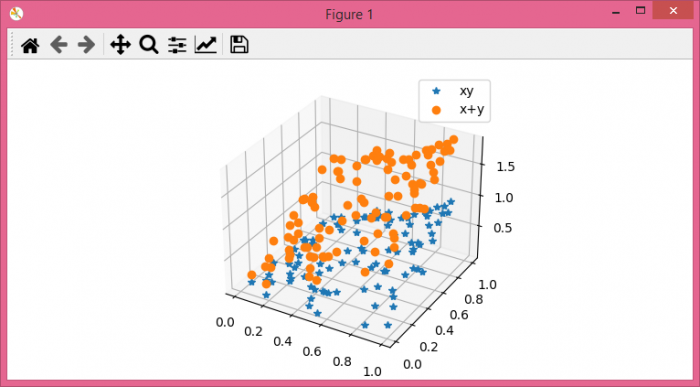# Add a legend in a 3D scatterplot with scatter() in Matplotlib

To add a legend in a 3D scatterplot with scatter() in matplotlib, we can take the following steps −

## Steps

• Set the figure size and adjust the padding between and around the subplots.

• Initialize a variable N to store the number of sample data.

• Create x and y data points; make z1 and z2 data points list.

• Add a subplot to the current figure, with projection='3d'.

• Plot the x, y and z1 data points using plot() points on 2d axes, with marker *.

• Plot the x, y and z2 data points using plot() points on 2d axes, with marker o.

• Place legend on the figure.

• To display the figure we can use show() method.

## Example

import matplotlib.pyplot as plt
import numpy as np

plt.rcParams["figure.figsize"] = [7.50, 3.50]
plt.rcParams["figure.autolayout"] = True

N = 100

x = np.random.rand(N)
y = np.random.rand(N)

z1 = [i*j for (i, j) in zip(x, y)]
z2 = [i+j for (i, j) in zip(x, y)]

axes = plt.subplot(111, projection='3d')

axes.plot(x, y, z1, "*", label="xy")
axes.plot(x, y, z2, "o", label="x+y")

plt.legend(loc="upper right")

plt.show()

## Output

It will produce the following output −